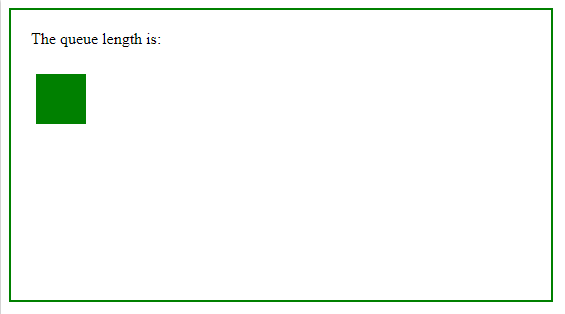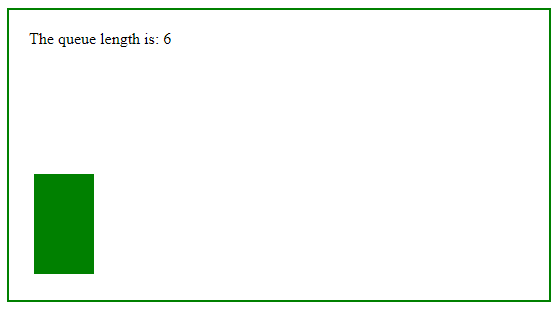Open in App
Not now

# jQuery | queue() with Examples

• Last Updated : 13 Feb, 2019

The queue() method is an inbuilt method in jQuery which is used to show the queue of functions to be executed on the selected elements. In a queue one or more function wait for run.

• The queue() method can be used with the dequeue() method.
• An element may have several queues. Generally there is only one default jQuery queue.

Syntax:

`\$(selector).queue(queue_name)`

Parameters: This method accepts single parameter queue_name which is optional. It is used to set the queue name.

Below example illustrates the queue() method in jQuery:

Example:

 ` ` `<``html``> ` `    ``<``head``> ` `        ``<``title``>The queue Method ` `        ``<``script` `src``= ` `        ``"https://ajax.googleapis.com/ajax/libs/jquery/3.3.1/jquery.min.js"``> ` `        `` ` `         `  `        ``` `        ``<``script``> ` `            ``\$(document).ready(function() { ` `                ``\$("p").click(function() { ` `                    ``var div = \$("div"); ` `      `  `                    ``div.animate({ ` `                        ``left: "+=200" ` `                    ``}, 2000); ` `                    ``div.animate({ ` `                        ``height: 200 ` `                    ``}, "slow"); ` `                    ``div.animate({ ` `                        ``width: 150 ` `                    ``}, "slow"); ` `                    ``div.animate({ ` `                        ``height: 100 ` `                    ``}, "slow"); ` `                    ``div.animate({ ` `                        ``width: 60 ` `                    ``}, "slow"); ` `                    ``div.animate({ ` `                        ``left: "-=200", ` `                        ``top: "+=100" ` `                    ``}, 2000); ` `      `  `                    ``\$("span").text(div.queue().length); ` `                ``}); ` `            ``}); ` `        `` ` `        ``<``style``> ` `            ``div { ` `                ``width: 50px; ` `                ``height: 50px; ` `                ``position: absolute; ` `                ``left: 35px; ` `                ``margin-top: 10px; ` `                ``background-color: green; ` `            ``} ` `              `  `            ``body { ` `                ``width: 500px; ` `                ``height: 250px; ` `                ``border: 2px solid green; ` `                ``padding: 20px; ` `            ``} ` `        `` ` `    `` ` `      `  `    ``<``body``> ` `        ``<``p``>The queue length is: <``span``> ` `         `  `        ``` `        ``<``div``> ` `    `` ` ` `

Output:
Before click on the paragraph element:After click on the paragraph element:My Personal Notes arrow_drop_up
Related Articles